## Target Marketing Campaign Using different Data Mining algorithms

We were given a dataset for Paralyzed Veterans of America (PVA) fundraising and we had to
develop various models to estimate the number of donors. To do this, we needed to interpret the
data and perform data cleaning, data transformations and ultimately build models using
Decis

## Data Illustration

Number of attributes: 480
Number of examples/observations: 23158
Independent variable: TARGET_B

Data Exploration: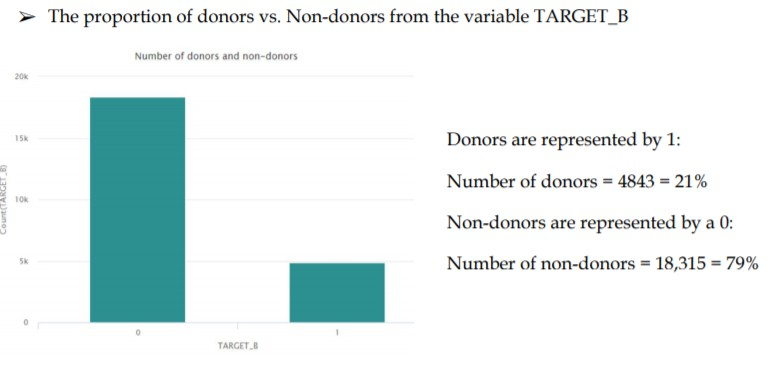In order to intuitively fish out the potentially relevant attributes we need to go through the data dictionary and understand the meaning of each and their relevance/contribution towards predicting the number of donors i.e TARGET_B. When we first scan through the dataset, we considered a number of variables to be potential important dependent variables. Here are some of them :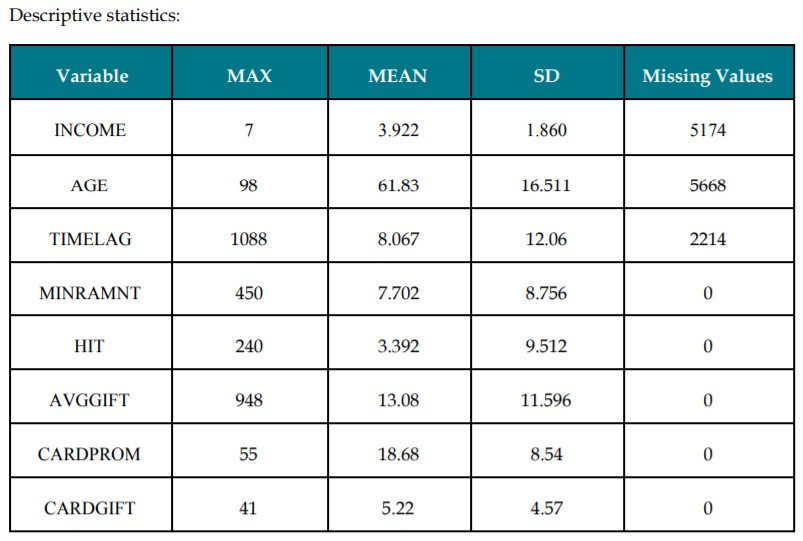By analyzing the data through the descriptive statistics shown above plotting relations with TARGET_B, we can understand the trends and relation to the target outcome variable. Other variables which we have discarded since we found them to be irrelevant and some had no contribution to the objective here. For example, the variable NUMCHILD which gives the number of children has 20,209 missing values i.e. there’s no information content in about 88% of the observations so we decided to exclude such variables from our prediction process. Further, we have implemented bivariate analysis with few variables and tried to find the relation with the target variable. Let’s see the graphical analysis for one of these: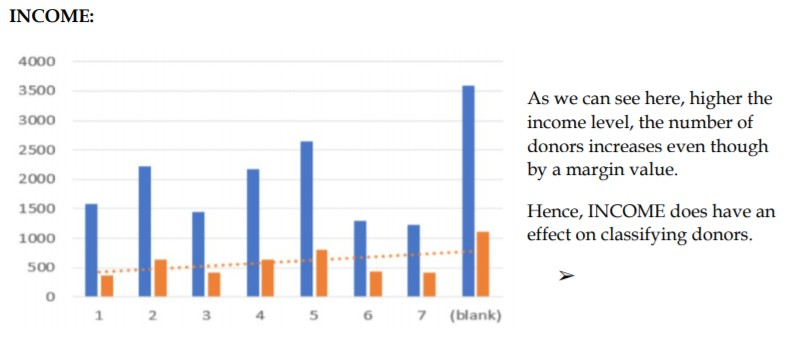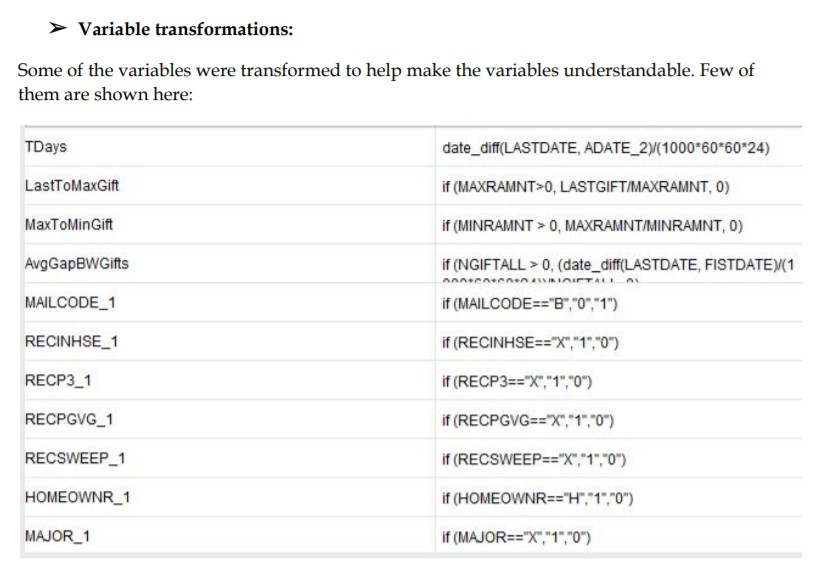### Omission of Variables

Understanding the relation of target variable with all 480 variables was turning out to be cumbersome and therefore we had to eliminate some attributes from the dataset.

We used the following ways to do so:

1. By intuition irrelevance: Some of the attributes had no relevance to our objective while some had only one factor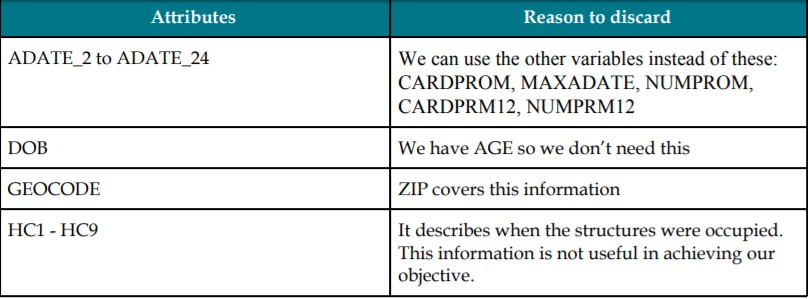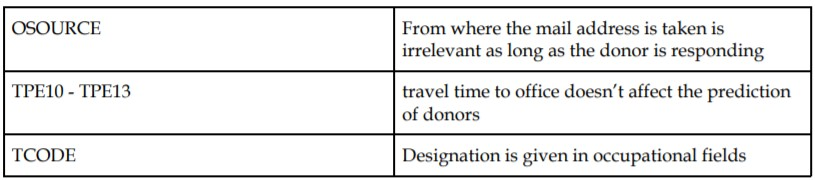2. Based on missing values: Too many missing values doesn’t help in information content evaluation. So we dropped such variables. Higher the number of missing values, the risk of imputing the values with some value and then that becoming the mode where a separate class gets created which doesn’t exist also increases. Some of the variables that we discarded are: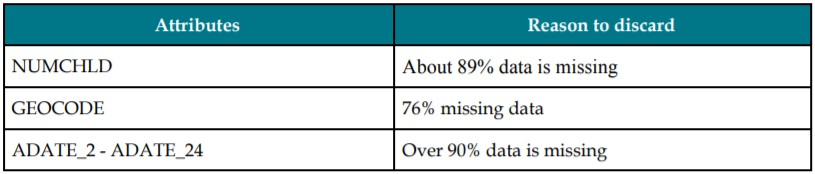On the other hand, there are cases where we kept a few variables although they had a lot of missing values.

PVA STATE - Over 98% data is missing but since it indicates if the donor belongs to the state server by the PVA chapter and so it might prove to be useful.

RFA variables - Varying missing data but it shows the designation which is related.

MAJOR - Over 90% missing data but we decided to keep it it since it shows the major donors.

Based on correlation - Some attributes that have high correlation among themselves have been discarded since this means there’ll be a large number of attributes causing redundancy. Using correlation matrix, the attributes where correlation 0.9 are removed like:

• RAMNT_3 to RAMNT_24
• RDATE_3 to RDATE_10

There’s very little correlation between these variables and hence discarded:

• MBCRAFT, MBGARDEN, MBBOOKS, MBCOLECT, MAGFAML, MAGFEM, MAGMALE, UBGARDN, PUBCULIN, PUBHLTH, PUBDOITY, PUBNEWFN, PUBPHOTO, PUBOPP

### Handling Misssing Values

After omitting variables, we need to clean the data by handling the various amounts of missing values for different attributes still in the dataset. Many of them have just empty values while some carry misinformation. Most importantly, we need to consider that missing values don’t always mean that data is actually missing for that record, so we cannot absolutely delete or ignore them. We can’t move forward with decision tree modelling or PCA analysis without complete data. So, we need to transform these variables to make them complete. The input values in the missing cases need to decided after precise analysis of the variable patterns and scenarios.

AGE - replace by mean value
TIMELAG - replaced by average time
GENDER - created a separate value as ‘Other’ - U

Missing values for numeric variables can be replaced with either the median or mean while those of categorical variables are treated as a newly created separate category.

### Variables considered for making data models

As described earlier based on descriptive stats and graphical analysis we think the below variables are important and could be considered for modeling:

• INCOME: Gives us an idea on how many people are earning and do they earn enough to be able to contribute as donation.
• AGE: Younger or older people are more likely to donate. Age distribution is a must. MAJOR: Shows us whether they have been major donors in the past.
• TIMELAG: Estimates the gap between two donations of a user. Helps us understand how often and after how long can they donate.
• HOMEOWNR: Do they own their own house or not? This shows us their assets to rely on.
• MDMAUD: This will highlight a potential donor from the rest with the bits of information that it provides.
• AVGGIFT: How much have they donated until now in the form of gifts. Historical information helps us predict the future amount too that they will likely donate.

### Data Reduction

Data reduction can be applied to reduce the data set that is much smaller in volume but still contain important information. We can do this in various ways like PCA (Principal components analysis), by choosing important attributes from decision tree models or random forests. Gini / Information gain can also help in deciding attributes or by checking the correlation matrix. We used the following steps to reduce data:
1. Intuitively remove attributes or based on missing values.
2. Removed correlated attributes.
3. Perform PCA analysis on subsets of attributes grouped categories explaining the variance in data.
4. Generate random forests to filter out the variables with least importance factor.

Principal Components Analysis (PCA)

We need to standardize the data and convert to numeric before starting the analysis. We have implemented the following four PCAs:

1. Interests_PCA: Here we used the 17 variables that depicted interests/hobbies of people which might help us in predicting the likeliness of them donating. Attributes used were BIBLE, BOATS, CARDS and so on...
2. Neighbourhood_PCA: 59 variables are used related to people’s nativity, ancestry. This helped us reduce the number of relevant attributes.
3. GOV_PCA: 6 variables like FEDGOV, LOCALGOV, etc grouped as the federal or government associates.
4. Promotion_PCA: 25 variables - RFA related values are considered to analyze based on the designation of the people in society.

Random forests and decision trees to determine which variables to include:

We can use decision trees to remove the attributes but here since the number of attributes are much more, these decision trees will need pruning. This will take a lot of time but even after this we cannot be confident about the results since there’s a risk of high variance. Hence, we decided to use random forest by weight. Random forest with gini index and number of trees = 500, depth = 100 helped us to decrease the attributes. Then we removed the attributes with weights 0.2 which decreased the variance too.

## Modelling

Partitioning

The dataset is partitioned into 60% training and 40% validation.

Consider the following classification techniques on the data:

• Decision Trees
• Logistic Regression, using Ridge and Lasso.
• Random forest
• Boosted trees

### Selection of Parameters for modelling

The dataset is divided into two sets - training and testing using 60-40 split and then used on different models created using decision trees, logistic regression, random forest and boosted trees. Earlier we removed the redundant variables using various experiments such as based on intuition, missing value percentage, correlation and much more to be able to predict the target variable i.e. the number of donors in the most precise and efficient manner. In order to do this, we have followed the below steps in RapidMiner:

• Remove useless attributes
• Generate new attributes
• Remove the related old attributes
• Map the missing values to something relatable
• Replace missing values in numeric and categorical variables
• PCA on interest variables - PCA on neighbourhood variables
• PCA on promotion related variables
• PCA on government or federal designation related variables
• Remove correlated variables
• Set the independent variable role
• Perform Random forest model with weight to reduce the dimensions
• Select attributes based on the weight reduction

For instance, if we perform the PCA analysis for interest variables and see the model performance then we will run the Neighbourhood_PCA and check the performance and so on until we get the final set of attributes.

At the end of this and some more rounds, we get the best group of variables that will be used for further modeling and ultimately predict the outcome variable - TARGET_B.

### Decision Trees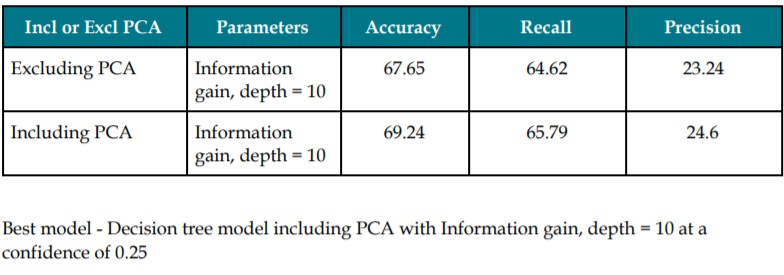### Logistic Regression (Ridge Lasso)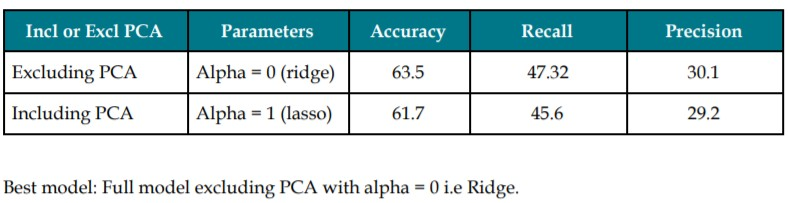Random Forest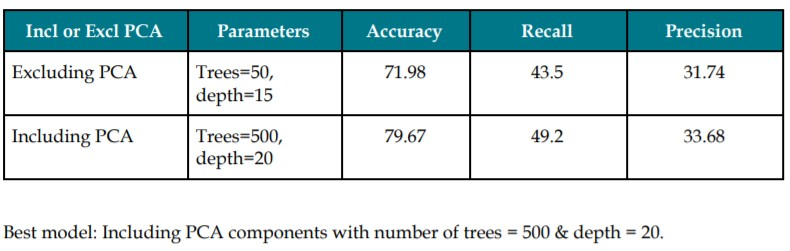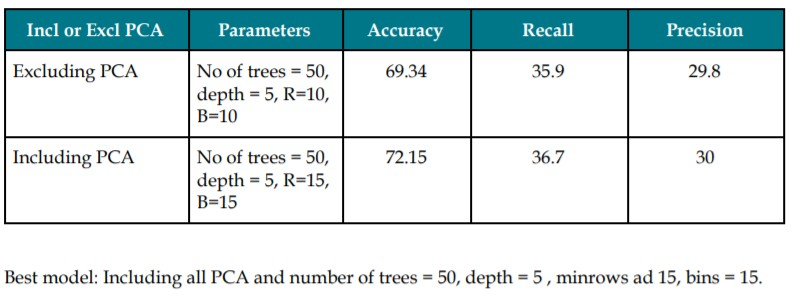## BEST MODEL

The best out of the above models is the Random Forest model including PCA, number of trees = 500 and depth = 20. This model has an accuracy of 79.67% and recall of 49.2. In order to reduce the risk of classifying a donor as a non-donor, we’ve got to choose a model with the highest recall.

The given dataset contains a higher proportion of donors to compensate for the low amount of donors as compared to the original dataset. This oversampling is done so that sufficient information can be retrieved from the donors to accurately construct the models. By ensuring that the proportion of donors to non-donors is nearly equal, the effect of bias on the model is reduced. We can be confident that the models will capture the patterns corresponding to both the classes (donors and non-donors) as a result of this weighted sampling. Since the response rate in the original dataset is only 5.1%, if we construct a model based on this, almost no relevant information regarding the interaction of the variables with donors can be acquired. This is because 95% of the dataset would’ve comprised of non-donors. If weighted sampling was not employed, the models based on training data would not have captured enough information to accurately predict the results. Also, as the dataset contains 480 variables, we need to accurately capture the effect each variable has on both donors and non-donors. As we are dealing with a weighted dataset, considering only ‘accuracy’ would not be a good decision. While calculating ‘accuracy’ we consider the total population. Since the total population parameter is weighted, the value of accuracy will be inaccurate. Instead, we can use ’recall’ and ‘precision’, as the calculations of these parameters do not include the weighted total population. From the information obtained, we can develop ROC curves and decide on the best model. In order to achieve Max Net Profit, we need to capture the maximum number of donors. Therefore, we concentrate on the recall and precision of the models in order to evaluate them.

Search
Categories